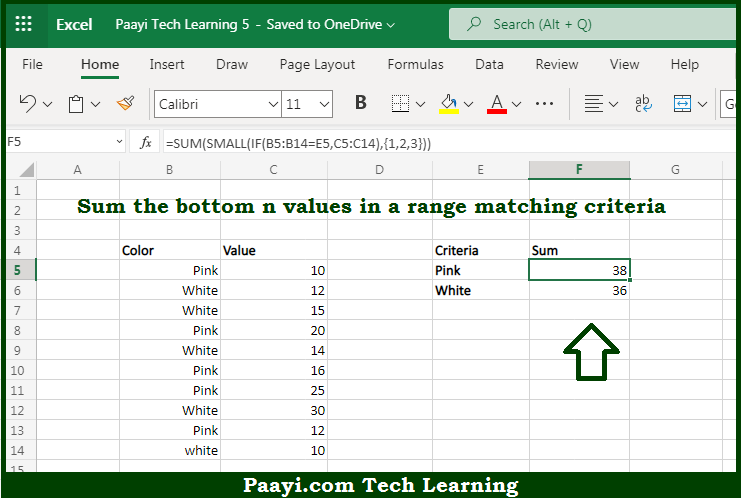# Learn How to SUM Bottom n Values With Criteria in Microsoft Excel

Written by | 0 Comments | 555 Views

In this article, you will learn how to SUM various things in Microsoft Excel using a single/combination(s) of functions. You will also know how to SUM Bottom n Values With Criteria and see the generic formula.

SUM Bottom n Values With Criteria in Microsoft Excel

The main purpose of this formula is to sum the bottom n values in a range matching criteria. Here we will learn how to sum bottom n values with provided criteria in the workbook in Microsoft Excel. That implies, with the help of a formula based on the SUM function you can able to sum the bottom n values in a range matching criteria. So, with the help of this formula, you can able to sum the bottom n values with provided criteria in the workbook in Microsoft Excel.

General Formula to SUM Bottom n Values With Criteria

{=SUM(SMALL(IF(range1=criteria,range2),{1,2,3,N}))}

The Explanation for the SUM Bottom n Values With CriteriaSo we know that with the help of the given formula above you can able sum the bottom n values in a range matching criteria. Here we will learn how to sum bottom n values with provided criteria in the workbook in Microsoft Excel. As we know that in the generic form of the formula provided here the range1 represents the range of cells compared to criteria. Similarly, the range2 contains numeric values from which bottom values are retrieved, and N represents "nth". So, with the help of this formula, you can able to sum the bottom n values with provided criteria in the workbook in Microsoft Excel.